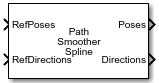# Path Smoother Spline

Smooth vehicle path using cubic spline interpolation

• Library:
• Automated Driving Toolbox## Description

The Path Smoother Spline block generates a smooth vehicle path, consisting of a sequence of discretized poses, by fitting the input reference path poses to a cubic spline. Given the input reference path directions, the block also returns the directions that correspond to each pose.

Use this block to convert a C1-continuous path to a C2-continuous path. C1-continuous paths include Dubins or Reeds-Shepp paths that are returned by path planners. For more details on these path types, see C1-Continuous and C2-Continuous Paths.

You can use the returned poses and directions with a vehicle controller, such as the Lateral Controller Stanley block.

## Ports

### Input

expand all

Reference poses of the vehicle along the path, specified as an M-by-3 matrix of [x, y, Θ] vectors, where M is the number of poses.

x and y specify the location of the vehicle in meters. Θ specifies the orientation angle of the vehicle in degrees.

Data Types: `single` | `double`

Reference directions of the vehicle along the path, specified as an M-by-1 column vector of 1s (forward motion) and –1s (reverse motion). M is the number of reference directions. Each element of RefDirections corresponds to a pose in the RefPoses input port.

Data Types: `single` | `double`

### Output

expand all

Discretized poses of the smoothed path, returned as an N-by-3 matrix of [x, y, Θ] vectors. N is the number of poses specified in the Number of output poses parameter.

x and y specify the location of the vehicle in meters. Θ specifies the orientation angle of the vehicle in degrees.

The values in Poses are of the same data type as the values in the RefPoses input port.

Driving directions of the vehicle at each output pose in Poses, returned as an N-by-1 column vector of 1s (forward motion) and –1s (reverse motion). N is the number of poses specified in the Number of output poses parameter.

The values in Directions are of the same data type as the values in the RefDirections input port.

You can use Directions to specify the reference path of a vehicle. You can also use Directions, along with CumLengths and Curvatures, to generate a reference velocity profile for the vehicle. See the Velocity Profiler block and the Automated Parking Valet in Simulink example.

Cumulative path lengths at each output pose in Poses, returned as an N-by-1 real-valued column vector. N is the number of poses specified in the Number of output poses parameter. Units are in meters.

You can use CumLengths, along with Directions and Curvatures, to generate a reference velocity profile for the vehicle. See the Velocity Profiler block and the Automated Parking Valet in Simulink example.

#### Dependencies

To enable this port, select the Show CumLengths and Curvatures output ports parameter.

Signed path curvatures at each output pose in Poses, returned as an N-by-1 real-valued column vector. N is the number of poses specified in the Number of output poses parameter. Units are in radians per meter.

You can use Curvatures, along with Directions and CumLengths, to generate a reference velocity profile for the vehicle. See the Velocity Profiler block and the Automated Parking Valet in Simulink example.

#### Dependencies

To enable this port, select the Show CumLengths and Curvatures output ports parameter.

## Parameters

expand all

Number of smooth poses to return in the Poses output port, specified as a positive integer. To increase the granularity of the returned poses, increase this parameter value.

Minimum separation between poses, in meters, specified as a positive real scalar. If the Euclidean (x, y) distance between two poses is less than this value, then the block uses only one of these poses for interpolation.

Sample time of the block, in seconds, specified as `-1` or as a positive real scalar. The default of `-1` means that the block inherits its sample time from upstream blocks.

Select this parameter to enable the CumLengths and Curvatures output ports.

• `Code generation` — Simulate the model using generated C/C++ code. The first time you run a simulation, Simulink® generates C/C++ code for the block. The C code is reused for subsequent simulations as long as the model does not change. This option requires additional startup time.

• `Interpreted execution` — Simulate the model using the MATLAB® interpreter. This option shortens startup time. In ```Interpreted execution``` mode, you can debug the source code of the block.

expand all

## Algorithms

• The path-smoothing algorithm interpolates a parametric cubic spline that passes through all input reference pose points. The parameter of the spline is the cumulative chord length at these points. 

• The tangent direction of the smoothed output path approximately matches the orientation angle of the vehicle at the starting and goal poses.

 Floater, Michael S. "On the Deviation of a Parametric Cubic Spline Interpolant from Its Data Polygon." Computer Aided Geometric Design. Vol. 25, Number 3, 2008, pp. 148–156.

 Lepetic, Marko, Gregor Klancar, Igor Skrjanc, Drago Matko, and Bostjan Potocnik. "Time Optimal Path Planning Considering Acceleration Limits." Robotics and Autonomous Systems. Vol. 45, Numbers 3–4, 2003, pp. 199–210.